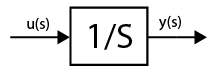# ISRO Scientist or Engineer Electrical 2015

Instructions

For the following questions answer them individually

Question 61

# OS that permits multiples programs to run simultaneously using single processoris referred asQuestion 62

# The cladding which surrounds the optic fiber line is usedQuestion 63

# Assuming zero initial condition, the response y(t) of the system given below to a unit step input u(t) isQuestion 64

# If the unit step response of a network is $$(1 - e^{-at})$$ then its unit impulse response isQuestion 65

# The solution of the differential equation $$\left(\frac{dy}{dx}\right) = ky, Y(0) = C$$ isQuestion 66

# The trigonometric Fourier series of an even function does not have theQuestion 67

# If $$x = \sqrt{-1}$$ then the value of $$x^x$$ isQuestion 68

# For the function $$f(t) = e^{\frac{-t}{r}}$$, the Taylor series approximation for $$t << \tau$$ isQuestion 69

# If the impedance of an AC circuit is $$10 \angle 60^\circ Ω$$ then resistance in the circuit isQuestion 70

# The dielectric strength of air under normal condition isOR